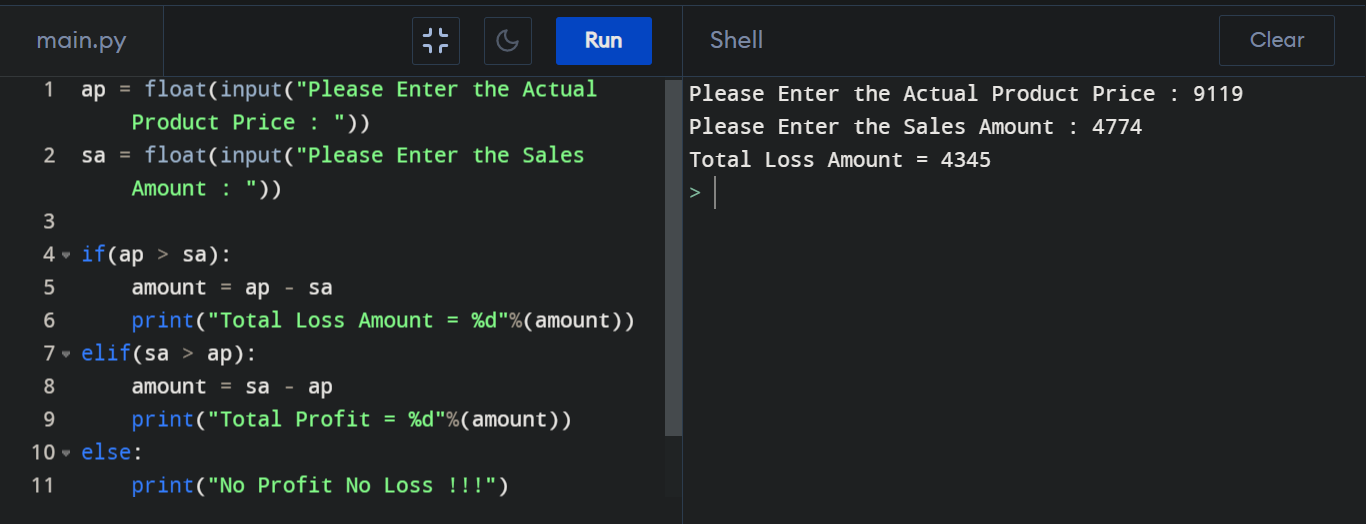## Python Program to Calculate Profit or Loss

How can we get the Profit or Loss amount? We can get the Profit or Loss amount by subtracting Sell price from Actual price.

#### Profit or Loss calculation using Python :

``````
ap = float(input("Please Enter the Actual Product Price : "))
sa = float(input("Please Enter the Sales Amount : "))

if(ap > sa):
amount = ap - sa
print("Total Loss Amount = %d"%(amount))
elif(sa > ap):
amount = sa - ap
print("Total Profit = %d"%(amount))
else:
print("No Profit No Loss !!!")

``````

#### Output :